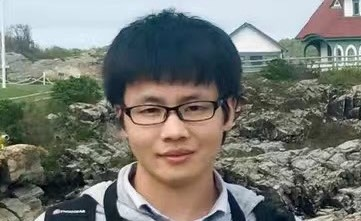# Events

## DMS Stochastic Analysis Seminar

Time: Apr 12, 2023 (01:10 PM)
Location: 352 Parker Hall

Details:Speaker: Dr. Xiong Wang, Johns Hopkins University

Title: Necessary and sufficient conditions to solve parabolic Anderson model with rough noise

Abstract: In this talk, we discuss necessary and sufficient conditions for the existence of $$n$$-th chaos of the solution to the parabolic Anderson model $$\frac{\partial}{\partial t}u(t,x) =\frac{1}{2}\Delta u(t,x)$$ $$+\, u(t,x)\dot{W}(t,x)$$, where $$\dot{W}(t,x)$$ is a fractional Brownian field with temporal Hurst parameter  $$H_0\ge 1/2$$ and spatial parameters $$H$$ $$=(H_1, \cdots, H_d)$$ $$\in (0, 1)^d$$. When $$d=1$$, we extend the condition on the parameters under which the chaos expansion of the solution is convergent in the mean square sense, which is both sufficient and necessary under some circumstances.

Short bio: Dr. Xiong Wang is a J.J. Sylvester Assistant Professor at Johns Hopkins University since August 2022. He received his Ph.D. from the University of Alberta in June 2022 under the supervision of Professor Yaozhong Hu. His research interests include stochastic processes, SPDEs, and data science.